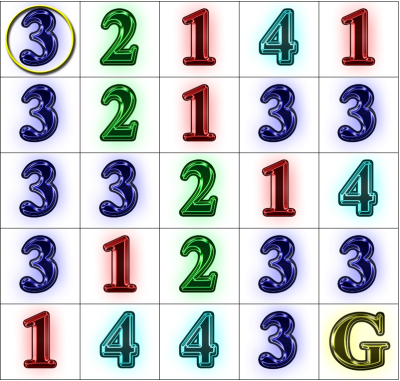# Restart Bandit

Rook Jumping Maze Instructions: Starting at the circled cell in the upper-left corner, find a path to the goal cell marked “G”.  From each numbered cell, one may move that exact number of cells horizontally or vertically in a straight line.  How many moves does the shortest path have?Solution (select to reveal): [19 moves: RDLRUDRLRLUDLDRRUDD]

Problem:  Use ε-greedy and softmax strategies to learn an approximately optimal restart period that maximizes generated maze utility per unit of computation.

"One-armed bandit" is a slang reference to a slot machine.  A well-known problem in psychology and machine learning is the n-armed bandit problem where the learner is faced with a set of single actions with variable payouts and must balance explorative actions that gain information about expected action utility, versus exploitative actions that use such information to maximize utility.

In this case, let us suppose that our goal is to create a Rook Jumping Maze generator that uses hill descent with random restarts in order to yield mazes with the longest solutions most efficiently.  Further, let us suppose that we value a maze with goal depth d twice as much as a maze with goal depth (d - 1).  Finally, suppose that computation has a uniform cost per iteration.  Let d = 0 for a maze without a solution.  Then we can measure the utility of a single hill descent of i iterations as 2d / i.

In this exercise, we will implement two simple techniques for learning the approximate best number of iterations i that maximizes the expected utility 2d / i  for hill descent.  Then one can restart after i iterations and expect to maximize expected utility of the generator per unit of computation.

For each method, we will create, in effect, a 6-armed bandit by allowing 6 choices for the number of iterations between each restart: 100, 200, 400, 800, 1600, 3200, that is, 100 * 2a, a in {0, ..., 5}.  Each method will learn the utility of actions by using a action selection strategy that allows explorative actions while largely taking actions that are expected to be superior.

The ε-greedy strategy for action selection chooses a random action with probability ε, and otherwise chooses an action with the maximum average utility experienced so far.  If ε = 1, this is purely explorative.  If ε = 0, this is purely exploitative.

The softmax strategy for action selection assigns a probability to the choice of each action a according to the normalized weight  e(U(a)/τ) for some given constant τ.  That is, we compute the sum of e(U(a)/τ) for all a, and divide each individual term of the sum by the sum to get the action probabilityA simple algorithm to compute a softmax action is as follows:

• For each action a,
• prob[a] =  e(U(a)/τ)
• sum = sum + prob[a]
• r = random number in [0, 1)
• For each action a,
• prob[a] = prob[a] / sum
• cumProb = cumProb + prob[a]
• if r < cumProb, return a

Evaluate ε-greedy strategy and softmax strategy for Hill Descent generation of Rook Jumping mazes.   Evaluate three different values of ε and τ for ε-greedy strategy and softmax strategy, respectively, for 10000 iterations each.  Print tables of the data collected for each 10000 iterations of learning.  (See transcript below for an example.)

Sample Transcript:

```e-greedy(epsilon=0.100000):
Iters	Avg. U		Total U		Count
100	29.093091	6400.480000	220
200	55.150983	9541.120000	173
400	133.123432	49655.040000	373
-->	800	154.603927	1368399.360000	8851
1600	123.704698	18432.000000	149
3200	71.111111	16640.000000	234

e-greedy(epsilon=0.500000):
Iters	Avg. U		Total U		Count
100	29.425384	24158.240000	821
200	62.412564	50866.240000	815
400	125.300579	108134.400000	863
-->	800	159.189108	933007.360000	5861
1600	130.904069	106163.200000	811
3200	71.226055	59046.400000	829

e-greedy(epsilon=1.000000):
Iters	Avg. U		Total U		Count
100	25.534548	42617.160000	1669
200	67.629684	113550.240000	1679
400	123.813365	211225.600000	1706
-->	800	157.323225	267292.160000	1699
1600	119.995910	197153.280000	1643
3200	70.797406	113559.040000	1604

softmax(tau=1.000000):
Iters	Avg. U		Total U		Count
100	0.000000	0.000000	0
200	0.000000	0.000000	0
400	0.000000	0.000000	0
-->	800	155.814144	1558141.440000	10000
1600	0.000000	0.000000	0
3200	0.000000	0.000000	0

softmax(tau=50.000000):
Iters	Avg. U		Total U		Count
100	34.719614	14408.640000	415
200	68.736124	35467.840000	516
400	126.309737	312111.360000	2471
-->	800	159.523264	633466.880000	3971
1600	121.644326	228326.400000	1877
3200	68.771840	51578.880000	750

softmax(tau=100.000000):
Iters	Avg. U		Total U		Count
100	31.231344	24391.680000	781
200	57.177993	61523.520000	1076
400	129.181492	275285.760000	2131
-->	800	158.914955	461171.200000	2902
1600	120.441905	227635.200000	1890
3200	74.624000	91041.280000	1220```

Questions:

1. Empirically, how does varying ε vary the behavior of ε-greedy strategy?  What happens for extreme high/low values of ε?
2. Empirically, how does varying τ vary the behavior of softmax strategy?  What happens for extreme high/low values of τ?
3. Which strategy do you prefer and why?
4. In the transcript above, we got lucky for the τ= 1 case.  As long as there are only positive utility experiences with an action, softmax strategy will tend not to deviate from it for low τ.  Similar behavior occurs for ε-greedy strategy with low ε.  One approach to this problem is to begin search with each action having a prior false experience with positive utility in order to encourage initial exploration.  Experiment with this approach and observe the results.  How should one choose the initial positive utility value?

Todd Neller